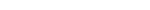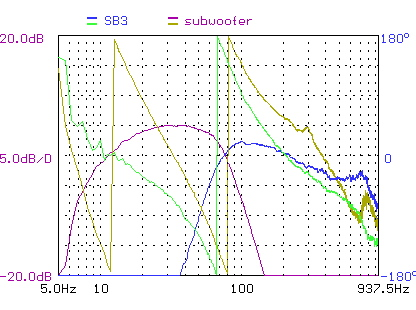HomeProductsTechnologyPlans & DIYOrderingReviewsAbout usFAQContact

 Subwoofer phase alignment

Calculating the delay required

1. First determine crossover point. In this example: 80 Hz

2. Next you need the phase shift of your main speaker relative to your subwoofer. In this example we will use the phase shift shown in Figure 1 below. The blue and cyan colors are the amplitude and phase response, respectively, of SB3 after 12db/oct HPF is applied. At 80hz, the phase shift is +135 degrees. The red and gold colors are the amplitude and phase response of the subwoofer, respectively, after 12db/oct LPF is applied. The subwoofer is -180 degrees (at 80hz) at this point. Both SB3 and subwoofer have crossover applied already. Keep in mind -180 and -225 degrees are same as +180 and +135 degrees, respectively. This means we need to add a phase shift of -45 degrees to the subwoofer to get phase aligned with the front speakers to -225 degrees to align with +135 degrees of SB3. 45 degrees is one eighth of a cycle.

3. Calculate the distance of one wavelength at the crossover point by the following formula:

wavelength = 344 / frequency(metres)

wavelength = 344 / 80

wavelength = 4.3m

4. Now convert phase shift into cycles

cycles = phase shift / 360

cycles = -45 /360

cycles = -0.125

5. Now multiply the number of cycles by the wavelength

In this case the distance compensation is -0.125 x 4.3 = -0.5m

In other words, the distance setting on the subwoofer should be adjusted so that we add this number to the physical distance. If your subwoofer is placed 4 metres from your listening position, then you should enter 3.5 m. The sign of distance adjustment is very important. By doing this, we trick the AVR to think the subwoofer is 0.5m closer and therefore it will delay subwoofer signal by an amount of time that sound travels 0.5m distance.

Some readers have questions about the relationship between the sign of the phase adjustment and subwoofer distance setting. First we need to recognize that phase adjustment implemented with analog circuits is always adding "negative phase angle" to the phase response plot. Even though on our plate amp, we have used 0 degree and 180 degrees on the decal, it really should have read as -180 degrees. So it is important to note "adding delay time in time domain" = "adding negative phase angle" = "subtracting physical distance in AVR".Figure 1 - NHT SB3 and subwoofer amplitude and phase response with crossovertop